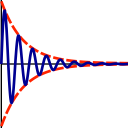Subsections

select.sn_ratioSynopsis

Select spins with signal to noise ratio higher or lower than the given ratio.

Defaults

select.sn_ratio(ratio=10.0, operation=`>', all_sn=True)

Keyword arguments

ratio: The signal to noise ratio to compare to.

operation: The comparison operation by which to select the spins.

all_sn: A flag specifying if all the signal to noise ratios per spin should match the comparison operator, of if just a single comparison match is enough.

Description

The comparison operation is the method which to select spins according to: operation(sn_ratio, ratio).

The possible operations are: `<':strictly less than, `<=':less than or equal, `>':strictly greater than, `>=':greater than or equal, `==':equal, `!=':not equal.

The `all_sn' flag default is True, meaning that if all of the spin's signal to noise levels evaluates to True in the comparison, the spin is selected.

Prompt examples

To select all spins with a signal to noise ratio higher than 10.0:

```[numbers=none]
relax> select.sn_ratio(ratio=10.0, operation='>')
```

```[numbers=none]
relax> select.sn_ratio(ratio=10.0, operation='>', all_sn=False)
```

The relax user manual (PDF), created 2019-06-14.